International Journal of Materials Science and Applications
Volume 5, Issue 3, May 2016, Pages: 125-128

First-Principles Calculation for Thermodynamic Properties of LiD

Lei Jie-Hong1, 2, Gu Yuqiu1

1Research Center of Laser Fusion, National Key Laboratory of Laser Fusion, CAEP, Mianyang, China

2Physics and Space Science of Institute, West China Normal University, Nanchong, China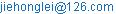(Lei Jie-Hong)

Lei Jie-Hong, Gu Yuqiu. First-Principles Calculation for Thermodynamic Properties of LiD. International Journal of Materials Science and Applications. Vol. 5, No. 3, 2016, pp. 125-128. doi: 10.11648/j.ijmsa.20160503.12

Received: April 20, 2016; Accepted: May 16, 2016; Published: May 19, 2016

Abstract: In this paper, the lattice parameter and bulk modulus of LiD at different pressures and temperatures are calculated by using the density functional theory method within the generalized gradient approximation (GGA). Through the quasi-harmonic Debye model, the thermodynamic properties of the LiD are predicted. The dependences of the normalized primitive cell volume V/V0 on pressure P, the variation of the thermal expansion coefficient α with pressure P and temperature T, as well as the dependences pf the heat capacity Cv on pressure P and temperature T are obtained systematically in the ranges of 0-100GPa and 0-2000K.

Keywords: Density Functional Theory, the Quasi-Harmonic Debye Model, Thermodynamic Properties

Contents

1. Introduction

Lithium hydride thin film is an ideal candidate of the fusion fuel in inertial confinement fusion . Lithium hydride is the lightest of metal hydrides with a high melting temperature of 688°C. It is thermodynamically stable in vacuum and inert atmospheres and is chemically more stable than pure lithium [2-3]. Moreover the isotope 6Li can react with neutrons to create tritium, which can increase the content of tritium in fusion reaction. Because of these properties, lithium hydride has the most extensive applied foreground in thermonuclear weapons.

Lithium hydride one of the simplest neutral heteropolar diatomic molecules, lightest heteronuclear diatomics and an important candidate in spectroscopic studies to demonstrate the breakdown of the Born-Oppenheimer approximation has long attracted the interest of spectroscopists and theoreticians [4-9].

It is well known that high-pressure and high –temperature studies play an important role in understanding the fundamental physical properties of condensed matter. The high pressure structural phase transformation from a typical metal at ambient pressure to an orthorhombic phase for LiD has been reported to occur at 50GPa . However, there are few literatures reporting on the thermodynamic properties for LiD at different pressures and temperatures.

In this paper, we investigate the thermodynamic properties of LiD at different pressures and temperatures through the density functional theory method, and the quasi-harmonic Debye model . The brief review of the theoretical methods is presented in Section 2. In Section 3, we make some discussion of calculated results.

2. Materials and Methods

In our calculations, the ultrasoft pseudopotentials introduced by Vanderbilt  for the interactions of the electrons with the ion cores is applied, together with the generalized gradient approximation (GGA)  for exchange-correlation functional in the scheme of Perdew-Burke-Ernzerhof (PBE) . The electronic wave functions are expanded in a plane wave basis set with an energy cut-off of 350eV. As for the Brillouin-zone k-point sampling, the 10×10×10 Monkhorst-Pack mesh  are employed, where the self-consistent convergence of the total energy is 10-6eV/Atom. All the electronic structure calculations are implemented through the CASTEP code [16,17].

In order to investigate the thermodynamic properties, we apply the quasi-harmonic Debye model, in which the non-equilibrium Gibbs function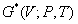can be written as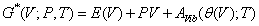(1)

where E(V) is the total energy per unit cell of the crystal, which can be determined from the electronic structure calculations. The term PV corresponds to the constant hydrostatic pressure condition.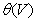is the Debye temperature, and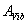is the vibrational Helmholtz free energy. It is customary to be written as [18, 19]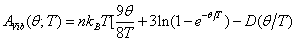(2)

Here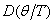represents the Debye integral, n is the number of atoms per formula unit. For an isotropic solid,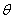is expressed by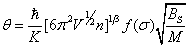(3)

Where M is the molecular mass per formula unit,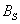is the adiabatic bulk modulus,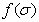andare given by Ref. .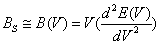(4)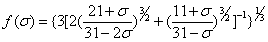(5)

Therefore, the non-equilibrium Gibbs functionas a function of (V; P, T) can be minimized with respect to volume V,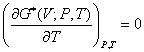(6)

Thus one could get the thermal equation of state (EOS) V (P, T) by solving Eq. (6). The heat capacity Cv, the isothermal bulk modulus BT, and the thermal expansion coefficient α are given by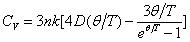(7)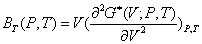(8)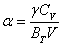(9)

where γ is the Grüneisen parameter, which is defined as(10)

One could calculate the thermodynamic properties of LiD at different pressures and temperatures from the calculated energy-volume data at T=0 and P=0. Applying there methods described above, the thermodynamic properties of some materials have successfully been investigated [18-20].

3. Results and Discussion

First of all, we set a series of different values of lattice constant to calculate the total energy E and the corresponding primitive cell volume V for the metal LiD at P=0GPa and T=0K. In Fig. 1, we present the E-V curves for several values of a. Then by fitting the calculated E-V curves to the natural strain EOS , we have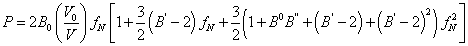(11)

where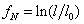, which may be written as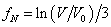for hydrostatic compression.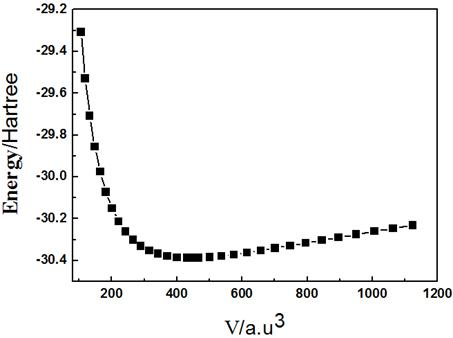Fig. 1. The energy-volume curves for different of a.

Now, we investigate the thermodynamic properties of LiD by applying the quasi-harmonic Debye mode described above. The normalized volume-pressure diagrams of LiD at the temperature ranges of 0-2000K are illustrated in Fig. 2. It is noted that, as the temperature T changes, the relative volume V/V0 of LiD doesn’t change at the same pressure. On the other hand, as the pressure P increases, the relative volume V/V0 of LiD decreases at a given temperature. The relationship between the bulk modulus B and pressure P at different temperatures are shown in Fig. 3. The results indicate that B increases with P at a given temperature, and at a given pressure, B increases with T. We illustrate the variations of the thermal expansion α with temperature and pressure in Fig. 4. It is shown that, for a given pressure, α increases rapidly with temperature at low temperature especially at zero pressure. As the pressure increases, the increase of α with temperature becomes smaller, especially at higher temperatures. α decreases drastically with the increase of pressure at a given temperature. The temperature of dependence of α is small at high pressure and high temperature.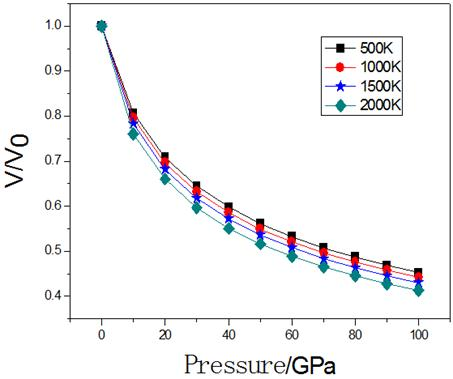Fig. 2. The normalized volume V/V0-P diagrams of LiD at the temperature ranges of 0-2000K.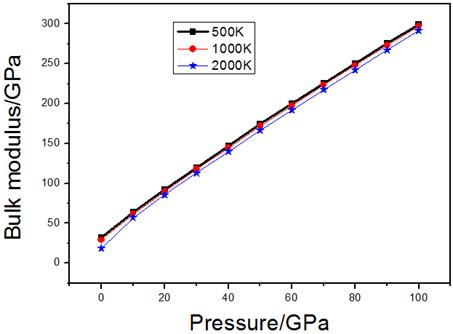Fig. 3. The relationships between bulk modulus B and pressure P at temperatures of 500, 1000, and 1500K.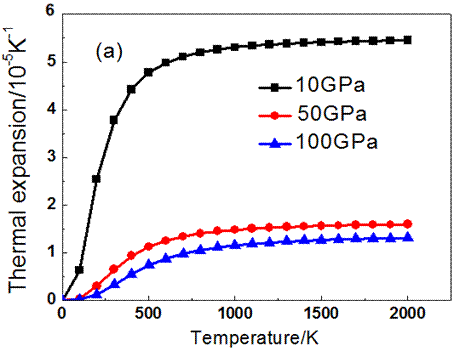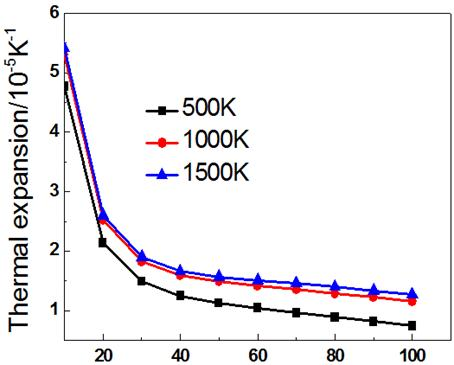Fig. 4. The thermal expansion versus pressure and temperature (a) and (b) for LiD.

The heat capacities of the metal LiD at various pressures and temperatures are plotted in Fig. 5, which shows that when T<1500K, the heat capacity CV is dependent on temperature and pressure. This is due to the anharmonic approximations of the Debye model here. However, under higher temperatures, the anharmonic effect on heat capacity CV is suppressed, and CV is very close to the Dulong-Petit limit.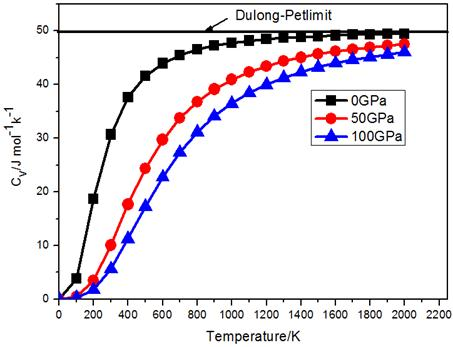Fig. 5. The heat capacity of LiD at various pressures and temperatures.

4. Conclusion

In summary, the thermodynamic properties of the metal LiD at different pressures and temperatures are investigated by using density functional theory method within the generalized gradient approximation (GGA). Applying the quasi-harmonic Debye model, we predict the thermodynamic properties of the metal LiD. The dependences of the relative volume V/V0 on pressure, the variations of the thermal expansion coefficient α and the CV with pressure and temperature have been obtain systematically in the ranges of 0-100GPa and 0-2000K.

References

1. R. Ren, A. L. Ortiz, T. Markmaitree, W. Osborn, and L Leon. Shaw. Stability of lithium hydride in argon and air [J]. J. Phys. Chem. B, 2006, 110: 10567-10575.
2. L. Schlapbach, A. Züttel, Hydrogen-storage materials for mobile applications [J]. Nature, 2001, 414: 353-358.
3. Ping Chen, Zhitao Xiong, Jizhong Luo, J Y Lin and K L Tan. Interaction of hydrogen with metal nitride and imides [J]. Nature, 2002, 420: 302-304.
4. C. Gatti, S. Polezzo, M. Raimondi and M Simonetta. Generalized self-consistent valence bond method for ground and excited potential energy surfaces [J]. Moecular. Physics, 1980, 41 (6): 1259-1279.
5. W. C. Stwalley, W. T. Zemke Spectroscopy and structure of the lithium hydride diatomic molecules and ions [J]. J. Phys. Chem. Ref. Data, 1993, 22 (1): 87-89.
6. Xiang-Jian Meng, Jian-Gong Chen, Hong-Juan Ye, Ping-xion Yang, Shan-ling Guo and Jun-hao Chu. Infrared reflection spectra and phonon modes of PbTiO3 polycrystalline thin film. J. Infrared Millim. Waves 18, No 5, 392–396 (1999).
7. C. R. Vidal, W. C. Stwalley, The A sigma-X sigma system of the isotopic lithium hudrides: the molecular constants, potential energy curves and their adiabatic corrections [J]. J. Chem Phys, 1982, 77: 883-898.
8. J. A. Coxon, The radial Hamiltonian operator for LiH X1∑+ [J]. J. Mol. Spectrosc, 1992, 152: 274-282 .
9. Zhi-F. Li, W. Lu, J. Infrared Millim. Waves, 22, No 1, 8–12 (2003).
10. V. E. Fortov, V. V. Yakushev, K L Kagan, et al. 2002 J. Phys. Condens. Matter 14 10809 .
11. Blanco M A, Francisco E and Luana V. 2004 Comput. Phys. Commun 158 57 .
12. Vanderbilt D. 1990 Phys. Rev. B 41 7892 .
13. Hammer B, Hansen L B and Norskov J K. 1999 Phys. Rev.B59 7413.
14. Perdew J P, Burke K and Ernzerh of M. 1996 Phys. Rev. Lett 77 3865 .
15. Monkhorst H J and Pack J D. 1976 Phys. Rev. B 13 5188 .
16. Payne M C, Teter M P, Allen D C, Arias T A and Joannopoulos J D. 1992 Rev. Mod. Phys 64 1045 .
17. Milan V, Winker B, White J A, Packard C J, Payne M C, Akhmatskaya E V and Nobes R H. 2002 Int. J. Quantum Chem 77 85.
18. Blanco M A, Pendás A M, Francisco E, Recio J M and Franco R. 1996 J.Mol Struct368 245 .
19. Flórez M, Recio J M, Francisco E, Blanco M A and Pendás A M. 2002 Phys. Rev. B 66 144112 .
20. Francisco E, Blanco M A and Sanjurjo G. 2001 Phys. Rev. B 63 094107 .
21. Wang Yanju, Tan Jiajin, Wang Yongliang and Chen Xiangrong. 2007 Chin. Phys. Soc 16 3046 .
22. Wang Chunlei, Yu Benhai, Huo Hailiang, Chen Dong and Sun Hai bin. 2009 Chin. Phys. Soc 18 1248 .
23. Hu Cuie, Zeng Zhaoyi, Cheng Yan, Chen Xiangrong and Cai Lingcang. 2008 Chin. Phys. Soc 17 3867.

 Contents 1. 2. 3. 4.
Article ToolsAbstractPDF(1205K)